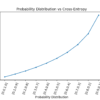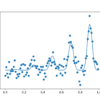# Archive | Probability## A Gentle Introduction to Logistic Regression With Maximum Likelihood Estimation

Logistic regression is a model for binary classification predictive modeling. The parameters of a logistic regression model can be estimated by the probabilistic framework called maximum likelihood estimation. Under this framework, a probability distribution for the target variable (class label) must be assumed and then a likelihood function defined that calculates the probability of observing […]## A Gentle Introduction to Linear Regression With Maximum Likelihood Estimation

Linear regression is a classical model for predicting a numerical quantity. The parameters of a linear regression model can be estimated using a least squares procedure or by a maximum likelihood estimation procedure. Maximum likelihood estimation is a probabilistic framework for automatically finding the probability distribution and parameters that best describe the observed data. Supervised […]## A Gentle Introduction to Maximum Likelihood Estimation for Machine Learning

Density estimation is the problem of estimating the probability distribution for a sample of observations from a problem domain. There are many techniques for solving density estimation, although a common framework used throughout the field of machine learning is maximum likelihood estimation. Maximum likelihood estimation involves defining a likelihood function for calculating the conditional probability […]## A Gentle Introduction to Cross-Entropy for Machine Learning

Cross-entropy is commonly used in machine learning as a loss function. Cross-entropy is a measure from the field of information theory, building upon entropy and generally calculating the difference between two probability distributions. It is closely related to but is different from KL divergence that calculates the relative entropy between two probability distributions, whereas cross-entropy […]## How to Calculate the KL Divergence for Machine Learning

It is often desirable to quantify the difference between probability distributions for a given random variable. This occurs frequently in machine learning, when we may be interested in calculating the difference between an actual and observed probability distribution. This can be achieved using techniques from information theory, such as the Kullback-Leibler Divergence (KL divergence), or […]## Information Gain and Mutual Information for Machine Learning

Information gain calculates the reduction in entropy or surprise from transforming a dataset in some way. It is commonly used in the construction of decision trees from a training dataset, by evaluating the information gain for each variable, and selecting the variable that maximizes the information gain, which in turn minimizes the entropy and best […]## A Gentle Introduction to Information Entropy

Information theory is a subfield of mathematics concerned with transmitting data across a noisy channel. A cornerstone of information theory is the idea of quantifying how much information there is in a message. More generally, this can be used to quantify the information in an event and a random variable, called entropy, and is calculated […]## A Gentle Introduction to Bayesian Belief Networks

Probabilistic models can define relationships between variables and be used to calculate probabilities. For example, fully conditional models may require an enormous amount of data to cover all possible cases, and probabilities may be intractable to calculate in practice. Simplifying assumptions such as the conditional independence of all random variables can be effective, such as […]## How to Implement Bayesian Optimization from Scratch in Python

In this tutorial, you will discover how to implement the Bayesian Optimization algorithm for complex optimization problems. Global optimization is a challenging problem of finding an input that results in the minimum or maximum cost of a given objective function. Typically, the form of the objective function is complex and intractable to analyze and is […]## How to Develop a Naive Bayes Classifier from Scratch in Python

Classification is a predictive modeling problem that involves assigning a label to a given input data sample. The problem of classification predictive modeling can be framed as calculating the conditional probability of a class label given a data sample. Bayes Theorem provides a principled way for calculating this conditional probability, although in practice requires an […]